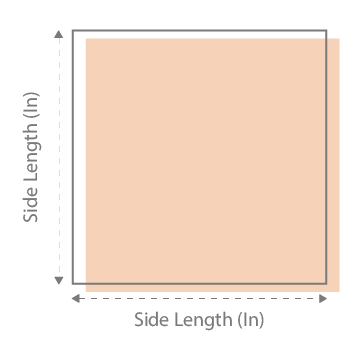X

## Select a Calculator

Please enter values in the input to get it convert into square feet then press "Calculate".

## Free calculator widget

You can put this calculator widget on your website, web page or blog.

Remember, the input can only be in feet (ft), inches (in), yards (yd), centimetres (cm), millimetres (mm) and metres (m) but never a combination of two different units!

Area = Side length x Side length
Length (drop down ft, in, yd, cm, mm, m)
Length (drop down ft, in, yd, cm, mm, m)
Price per unit (drop down ft2, in2, yd2, cm2, mm2, m2)# Understanding the Square footage:

To understand how to calculate square footage we must first begin with the definition of area. An area is the size of a two-dimensional surface. The area of a square is the space contained within a set of lines. These lines should be measured in feet (ft) for square footage calculations and if needed, converted to inches (in), yards (yd), centimetres (cm), millimetres (mm) and metres (m).

Area of a Square= Side length x Side length
Answer = (Side length x Side length) square area
Abbreviations of unit area: ft2, in2, yd2, cm2, mm2, m2

Where do you need it in daily life?

Our SQFT Calculator helps you calculate the area required for roofing shingles, landscaping for a garden, tiling, flooring space, carpeting and wallpaper. There is even a Siding Square Footage Calculator so you can estimate House Siding costs without any hassle.

What measurements do you need?

You need to know the length of one side of the area you wish to measure in either feet (ft), inches (in), yards (yd), centimetres (cm), millimetres (mm) or metres (m).

What can you calculate with this tool?

You can calculate the area in square feet, in square inches, square yards, square centimetres, square millimetres and square meters. Yes, it is that awesome.

Our calculator gives you the option of calculating the exact cost of materials. All you have to do is enter the price per unit area and voila, you have the total cost of materials in a single click!

Conversion factors:

To convert among square feet, square inches, square yards, square centimetres, square millimetres and square meters you can utilize the following conversion table.

 Square feet to square yards multiply ft2 by 0.11111 to get yd2 Square feet to square meters multiply ft2 by 0.092903 to get m2 Square yards to square feet multiply yd2 by 9 to get ft2 Square yards to square meters multiply yd2 by 0.836127 to get m2 Square meters to square feet multiply m2 by 10.7639 to get ft2 Square meters to square yards multiply m2 by 1.19599 to get yd2 Square meters to square millimetres multiply the m2 value by 1000000 to get mm2 Square meters to square centimetres multiply the m2 value by 10 000 to get cm2 Square centimetres to square metres multiply the cm2 value by 0.0001 to get mm2 Square centimetres to square millimetres multiply the cm2 value by 100 to get mm2 Square millimetres to square centimetres multiply the mm2 value by 0.000001 to get cm2 Square millimetres to square metres multiply the mm2 value by 1000000 to get m2